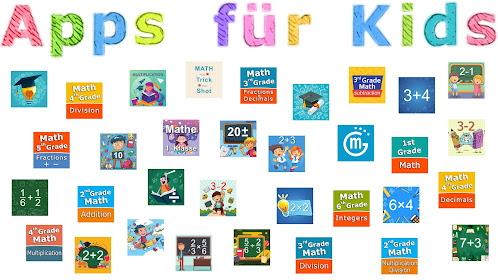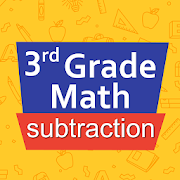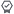Ages 9-12

# Third grade Math - SubtractionEveryone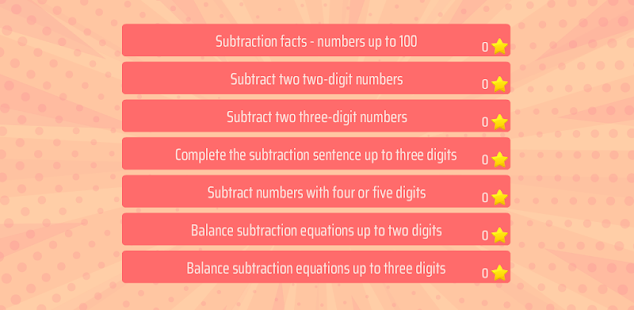Intuitive interface powered by handwriting input and a fun and engaging mini game in addition to a regular math trainer mode make our app stand out from the crowd of generic math learning apps.

With Third grade Math - Subtraction you can practice and improve the following math skills:
- Subtraction facts - numbers up to 100
- Subtract two two-digit numbers
- Subtract two three-digit numbers
- Complete the subtraction sentence up to three digits
- Subtract numbers with four or five digits
- Balance subtraction equations up to two digits
- Balance subtraction equations up to three digits
Collapse

## Reviews

Review policy and info

Eligible for Family Library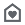Eligible if bought after 7/2/2016. Learn More
Updated
June 6, 2020
Size
Varies with device
Installs
500+
Current Version
Varies with device
Requires Android
Varies with device
Content Rating
Everyone
Permissions
Offered By
Sergey Malugin